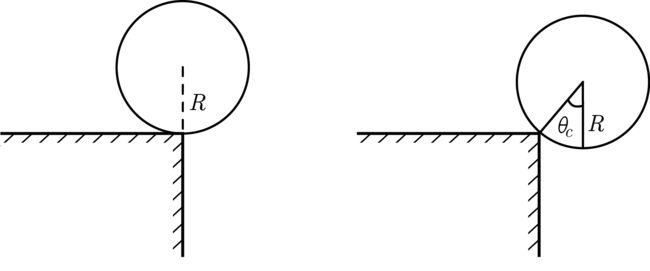# A very Edgy Problem!A cylinder is kept on the edge of a very rough table such that the edge and the axis of the cylinder lie on the same vertical plane. At that instant, there occurs some disturbance that produce slight push on the cylinder. If

I: $\theta_c$ is the angle through which the cylinder rotates before leaving the plane of the table

II: $v_{cm}$ is the speed of the centre of mass of the cylinder just before leaving the plane of the table

III: $c$ is the ratio of the translation to rotational kinetic energies of the cylinder when its centre of mass is in the horizontal line of the edge.

Evaluate $\Large 7\cos\!\theta_c + v_{cm} + c$.

Details and Assumptions

• Sufficient friction is present so that even a very small displacement causes rotation without slipping.

• Radius of the cylinder $R = 0.7\text{ m}$.

• Take $g = 10\text{ ms}^{-2}$.

×